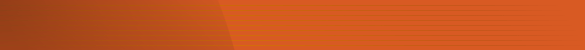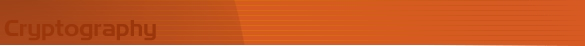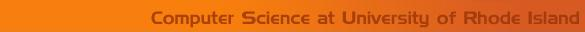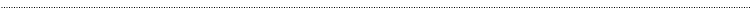Home Page Introduction Classical Ciphers Enigma Machine DES Key Agreement PGP R.S.A. Number Theory Other LinksClassical CryptographyShift Cipher

History and Description

In the first century B.C., Julius Caesar developed the shift cipher. Based on a shift key, each letter in the alphabet is replaced by the new shifted letter. The message which is enciphered by replacing the corresponding new letter is called plaintext, and the new message which is produced by the substitution is called ciphertext. The key gives the number of letters the alphabet is shifted between plaintext and ciphertext.

Example

Encipher
Given: The shift key is 5
Plaintext: I AM A STUDENT

The corresponding ciphertext letter is computed for each letter in the plaintext as follows
I + Shift(5) = N

A + Shift(5) = F
M + Shift(5) = R

A + Shift(5) = F

S + Shift(5) = X
T + Shift(5) = Y
U + Shift(5) = Z
D + Shift(5) = I
E + Shift(5) = J
N + Shift(5) = S
T + Shift(5) = Y

Therefore, the ciphertext is NFRFXYZIJSY.

Decipher
We decipher NFRFXYZIJSY. by computing the corresponding plaintext letter for each letter in the ciphertext as follows
N - Shift(5) = I

F - Shift(5) = A
R - Shift(5) = M

F - Shift(5) = A

X - Shift(5) = S
Y Shift(5) = T
Z - Shift(5) = U
I - Shift(5) = D
J - Shift(5) = E
S - Shift(5) = N
Y - Shift(5) = T

Therefore, the plaintext is IAMASTUDENT.